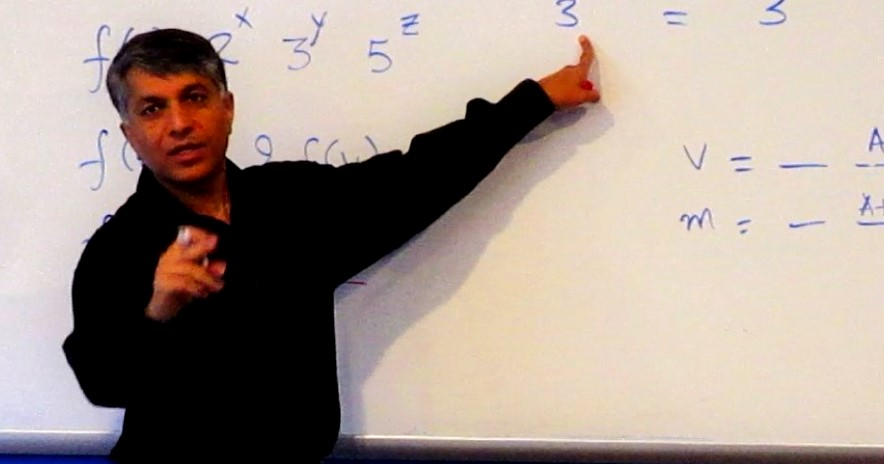GMATTM Prep Classes
512.797.9525

# GMAT Math Foundations

The GMAT quant section of Austin GMAT Review's course begins with a 6-hour Math Foundations class. The class covers fundamental arithmetic and algebra to allow you to brush up on math basics and prepare you for the more advanced GMAT math topics.GMAT Math Foundations class is included with all of our course packages.

#### Are Your Math Skills Rusty: Yes or No?

Just for fun, take the 10-minute self-assessment quiz below. As on the GMAT, you may use a pencil and paper but not a calculator.

Good luck on your quiz! The answer sheet is at the end.

#### Basic Math Assessment Test

(0.5)3 =
Oops! Something went wrong while submitting the form.
Correct!
Sorry, the correct answer is 0.125.10% of 150 =
Oops! Something went wrong while submitting the form.
Correct!
Sorry, the correct answer is 15.The square root of 0.4 is approximately
Oops! Something went wrong while submitting the form.
Correct!
Sorry, the correct answer is 0.6.For any real number X, the expression X3 + X3 =
Oops! Something went wrong while submitting the form.
Correct!
Sorry, the correct answer is 2x3.If X/5 is subtracted from X/3, the answer is
Oops! Something went wrong while submitting the form.
Correct!
Sorry, the correct answer is 2X/15.If M monkeys share a total of B bananas equally, how many bananas would each monkey get?
Oops! Something went wrong while submitting the form.
Correct!
Sorry, the correct answer is B/M.In triangle ABC, if angle A is 120 degrees, then angle B cannot be
Oops! Something went wrong while submitting the form.
Correct!
Sorry, the correct answer is 70 degrees.What is the maximum possible straight line distance between any two points on a circle of radius 1?
Oops! Something went wrong while submitting the form.
Correct!
Sorry, the correct answer is 2.What is the area of a square whose perimeter is 4?
Oops! Something went wrong while submitting the form.
Correct!
Sorry, the correct answer is 1.If u > t, r > q, s > t, and t > r, which of the following must be true?

I. u > s
II. s > q
III. u > r
Oops! Something went wrong while submitting the form.
Correct! And the quiz is complete.
Sorry, the answer is II and III. And the quiz is complete.Here is the answer sheet, but remember that you won't be allowed to bring notes into the GMAT exam!
Q1. (0.5)3 =
A1. 0.125
Q2. 10% of 150 =
A2. 15
Q3. The square root of 0.4 is approximately
A3. 0.6
Q4. For any real number X, the expression X3 + X3 =
A4. 2X3
Q5. If X/5 is subtracted from X/3, the answer is
A5. 2X/15
Q6. If M monkeys share a total of B bananas equally, how many bananas would each monkey get?
A6. B/M
Q7. In triangle ABC, if angle A is 120 degrees, then angle B cannot be
A7. 70 degrees
Q8. What is the maximum possible straight line distance between any two points on a circle of radius 1?
A8. 2
Q9. What is the area of a square whose perimeter is 4?
A9. 1
Q10. If u > t, r > q, s > t, and t > r, which of the following must be true?
I. u > s
II. s > q
III. u > r
A10. II and III
Oops! Something went wrong while submitting the form.
You've Got Options.

# Ultra Comprehensive

98 Hours of GMAT Prep
Master the GMAT